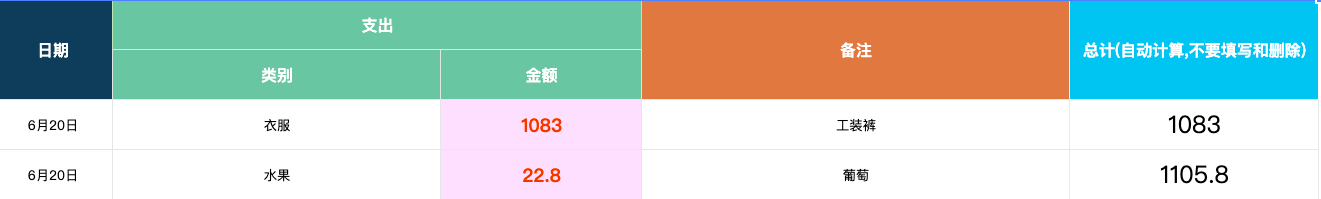0%### 1. 第一行到当前行求和

`=SUM(INDIRECT("A1:A"&ROW()))`

### 4. IF 函数

=IF(1>2,”判断真”,”判断假”)

### 7. 如果前面有值, 后面自动计算, 否则显示空

• 总金额

`=IF(ISBLANK(C4),"",SUM(INDIRECT("C4:C"&ROW())))` // C4是第一个数据, 对下面的列应用样式